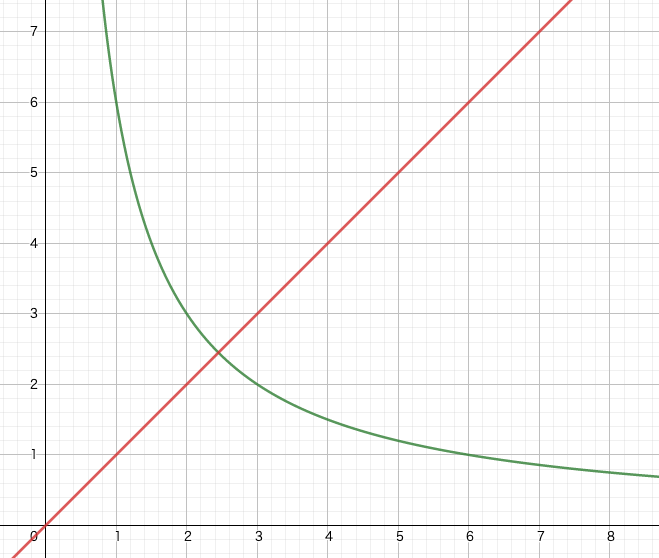# Preface (Introduction)

I would like to solve the other issue, Google's classified ads, with Ruby.

What is Google Job Ads?This is the picture above. Translated into Japanese, it is {the first consecutive 10-digit prime number in the value of \$ e \$} .com.

Now, let's solve it using Ruby.

Ruby

## Read the value of e

\$ e \$ is called the Napier number, and its definition is

Expressed as a convergence sequence, \$ e = \ lim_ {x \ to \ infinity} \ left (1 + \ frac {1} {x} \ right) ^ x \$

Expressed in calculus, \$ e = \ sum_ {n = 0} ^ \ infinty \ frac {1} {n!} \$.

\$ e \$ is an irrational number, but this time we will handle up to 200 digits.

``````2.71828182845904523536028747135266249775
7247093699959574966967627724076630353547
5945713821785251664274274663919320030599
2181741359662904357290033429526059563073
81323286279434907632338298807531952510190
``````

Save this value in `exp.txt` so that it loads every time you run the code.

``````def read_exp
return exp.delete('.')
end
``````

Reference: Number of Napiers & # x2013; Wikipedia

## Primality test

Create a function to determine if the argument n is a prime number.

For n to be a prime number, it must be indivisible by all numbers from 2 to n-1. Therefore, find the remainder of numbers from 2 to n-1 and create a function called a prime number if all of them are not 0.

``````def prime? n
for i in 2..n-1 do
return false if n % i == 0
end
return true
end
``````

However, this would be a wasteful calculation, so the upper limit of iteration is \$ \ sqrt {n} + 1 \$.

Here's an explanation of why this is done. In the figure below, green represents the first quadrant of \$ xy = 6 \$ and red represents \$ y = x \$.At this time, the green line is axisymmetric with the red line as the axis. In other words, with the intersection \$ (x, y) = (\ sqrt {6}, \ sqrt {6}) \$ of the two graphs as the boundary, the order is reversed, but the same numbers are multiplied.
In that case, we only need to check half, so the range for primality test should be 2 ~ \$ \ sqrt {n} + 1 \$.

Also, since all prime numbers that exceed 2 are odd numbers, skip two to find the remainder.
``````def prime? n
i = 2
return true if n == 2
return false if n % i == 0
i += 1
max = Integer.sqrt(n) + 1
while i <= max do
return false if n % i == 0
i += 2
end
return true
end
``````

Code

Now, combine the existing functions to complete the program.

#### `exp_prime.rb`

``````
return exp.delete('.')
end

def prime? n
i = 2
return true if n == 2
return false if n % i == 0
i += 1
max = Integer.sqrt(n) + 1
while i <= max do
return false if n % i == 0
i += 2
end
return true
end

require "./assert_equal"
def test
expected = [2,3,5,7]
results = []
[*2..10].each do |num|
results << num if prime?(num)
end
assert_equal(expected, results)
end

def main
for i in 0..exp.length-10 do
next if exp[i] == '0'
exp10 = exp[i..i+9].to_i
if prime?(exp10)
puts exp10
break
end
end
end

if \$PROGRAM_NAME == __FILE__
main()
end
``````

If you move this

``````> ruby exp_prime.rb
-> 7427466391
``````

Superbly, it returned a prime number.

# Similar

Congratulations. You've made it to level 2. Go to www.Linux.org and enter Bobsyouruncle as the login and the answer to this equation as the password.

``````f(1)=7182818284
f(2)=8182845904
f(3)=8747135266
f(4)=7427466391
f(5)=__________
``````

Since the value of f (4) is equal to the value given earlier, it seems likely that this is also the value of \$ e \$. However, I don't know what else they have in common.

I was worried, so I gg. It seems that the common point is that the total number of each digit is 49.

Now, let's create a program based on that information.

#### `google_recruit.rb`

``````
require './exp_prime'

def main
cnt = 0
for i in 0..exp.length-10 do
sum = 0
for j in 0..9 do
sum += exp[i+j].to_i
end
if sum ==49
cnt += 1
puts "f(" + cnt.to_s + ") = " + exp[i..i+9]
end
end
end

if \$PROGRAM_NAME == __FILE__
main()
end
``````

The output of this program is

``````f(1) = 7182818284
f(2) = 8182845904
f(3) = 8747135266
f(4) = 7427466391
f(5) = 5966290435
f(6) = 2952605956
``````

Therefore, the answer is `5966290435`.